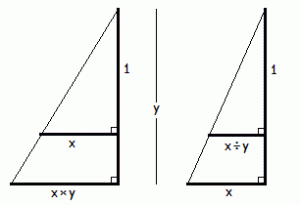# Constructions

Suki Tekverk, a summer intern, spent the last two weeks here studying APL. She will be a high school senior next September and is adept in math, so in addition to APL we also considered some math problems, proofs, and proof techniques, including the following:

Given line segments `x` and `y`, construct (using compass and straight-edge) line segments for the following values:
`   x+y`
`   x-y`
`   x×y`
`   x÷y`

The first two are immediate. Constructing the last two are straightforward if you are also given `1` (or some other reference length from which to construct `1`). Can you construct `x×y` and `x÷y` without `1`?

Constructing `x÷y` is impossible if you are not given `1` : From `x` and `y` alone you can not determine how they compare to `1`. If you can construct `x÷y`, then you can construct `x÷x` and therefore relate `x` and `y` to `1`, contradicting the previous statement.

Can you construct `x×y` without `1`? I got stuck (and lazy) and posed the question to the J Chat Forum, and received a solution from Raul Miller in less than half an hour. Miller’s proof recast in terms similar to that for `x÷y` is as follows:

From `x` and `y` alone you can not determine how they compare to `1`. If you can construct `x×y`, then you can construct `x×x`, whence:
`   `if `x<x×x` then `x>1`
`   `if `x=x×x` then `x=1`
`   `if `x>x×x` then `x<1`
I last thought about this problem in my first year of college many decades ago. At the time Norman M. (a classmate) argued that there must be a `1` and then did the construction for `x÷y` using `1`. I recall he said “there must be a 1” in the sense of “`1` has to exist if `x` and `y` exist” rather than that “you have to use a `1` in the construction” or “you can not construct `x÷y` without using `1`”. I don’t remember what we did with `x×y`; before Miller’s message I had some doubt that perhaps we were able to construct `x×y` without using `1` all those years ago. (Norman went on to get a Ph.D. at MIT and other great things.)